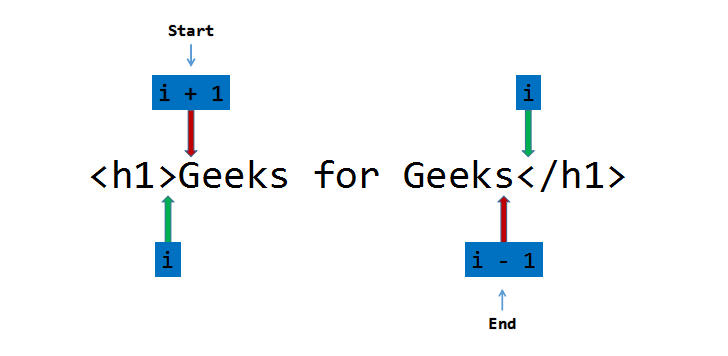Open in App
Not now

# HTML Parser in C/C++

• Difficulty Level : Hard
• Last Updated : 14 Jul, 2021

HTML Parser is a program/software by which useful statements can be extracted, leaving html tags (like <h1>, <span>, <p> etc) behind.

Examples:

Input: <h1>Geeks for Geeks</h1>
Output: Geeks for Geeks
Explanation- <h1> and </h1> are opening and closing heading tags, so they got parsed leaving “Geeks for Geeks” as the output.

Input: <p>    Geeks for Geeks</p>
Output: Geeks for Geeks
Explanation- <p> and </p> are opening and closing paragraph tags, so they get parsed and the parser ignores space character, leaving “Geeks for Geeks” as the output.

Approach: Let the input string be S of size N. Follow the steps below to solve the problem:

• Declare two variables, start and end to point to the starting and ending point of the statement.
• Traverse the string, S uses the variable i and if S[i] is equal to ‘>’, update the start variable to i+1 and break out of the loop.
• Remove the blank spaces from the start by running a loop while S[start] is equal to ‘ ‘, and increment the start variable by 1 in each iteration.
• Again, traverse the string, S from start using the variable i and if S[i] is equal to ‘<‘, update the end to i-1 and break out of the loop.
• Run a loop and print the characters of the string S in the range [start, end].Below is the implementation of the above approach in C language:

 `// C program for the above approach`` ` `#include ``#include ``#include `` ` `// Function to parse the HTML code``void` `parser(``char``* S)``{``    ``// Store the length of the``    ``// input string``    ``int` `n = ``strlen``(S);``    ``int` `start = 0, end = 0;``    ``int` `i, j;`` ` `    ``// Traverse the string``    ``for` `(i = 0; i < n; i++) {``        ``// If S[i] is '>', update``        ``// start to i+1 and break``        ``if` `(S[i] == ``'>'``) {``            ``start = i + 1;``            ``break``;``        ``}``    ``}`` ` `    ``// Remove the blank spaces``    ``while` `(S[start] == ``' '``) {``        ``start++;``    ``}`` ` `    ``// Traverse the string``    ``for` `(i = start; i < n; i++) {``        ``// If S[i] is '<', update``        ``// end to i-1 and break``        ``if` `(S[i] == ``'<'``) {``            ``end = i - 1;``            ``break``;``        ``}``    ``}`` ` `    ``// Print the characters in the``    ``// range [start, end]``    ``for` `(j = start; j <= end; j++) {``        ``printf``(``"%c"``, S[j]);``    ``}`` ` `    ``printf``(``"\n"``);``}`` ` `// Driver Code``int` `main()``{``    ``// Given Input``    ``char` `input1[] = ``"

This is a statement

"``;``    ``char` `input2[] = ``"

This is a statement with some spaces

"``;``    ``char` `input3[] = ``"

This is a statement with some @ #\$ ., / special characters

"``;`` ` `    ``printf``(``"Parsed Statements:\n"``);`` ` `    ``// Function Call``    ``parser(input1);``    ``parser(input2);``    ``parser(input3);`` ` `    ``return` `0;``}`

Output:

```Parsed Statements:
This is a statement
This is a statement with some spaces
This is a statement with some @ #\$ ., / special characters
```

Below is the implementation of the above approach in C++ language:

 `// C++ program for the``// above approach``#include ``using` `namespace` `std;`` ` `// Function to parse the``// HTML code``void` `parser(``char``* S)``{``    ``// Store the length of the``    ``// input string``    ``int` `n = ``strlen``(S);``    ``int` `start = 0, end = 0;`` ` `    ``// Traverse the string``    ``for` `(``int` `i = 0; i < n; i++) {``        ``// If S[i] is '>', update``        ``// start to i+1 and break``        ``if` `(S[i] == ``'>'``) {``            ``start = i + 1;``            ``break``;``        ``}``    ``}`` ` `    ``// Remove the blank space``    ``while` `(S[start] == ``' '``) {``        ``start++;``    ``}`` ` `    ``// Traverse the string``    ``for` `(``int` `i = start; i < n; i++) {``        ``// If S[i] is '<', update``        ``// end to i-1 and break``        ``if` `(S[i] == ``'<'``) {``            ``end = i - 1;``            ``break``;``        ``}``    ``}`` ` `    ``// Print the characters in the``    ``// range [start, end]``    ``for` `(``int` `j = start; j <= end; j++) {``        ``cout << S[j];``    ``}`` ` `    ``cout << endl;``}`` ` `// Driver Code``int` `main()``{``    ``// Given Input``    ``char` `input1[] = ``"

This is a statement

"``;``    ``char` `input2[] = ``"

This is a statement with  some spaces

"``;``    ``char` `input3[] = ``"

This is a statement with some @ #\$ ., / special characters

"``;`` ` `    ``cout << ``"Parsed Statements:\n"``;`` ` `    ``// Function Call``    ``parser(input1);``    ``parser(input2);``    ``parser(input3);``    ``return` `0;``}`

Output:

```Parsed Statements:
This is a statement
This is a statement with  some spaces
This is a statement with some @ #\$ ., / special characters
```

Time Complexity: O(N)
Auxiliary Space: O(1)

Note: This program parses only one statement at a time.

My Personal Notes arrow_drop_up